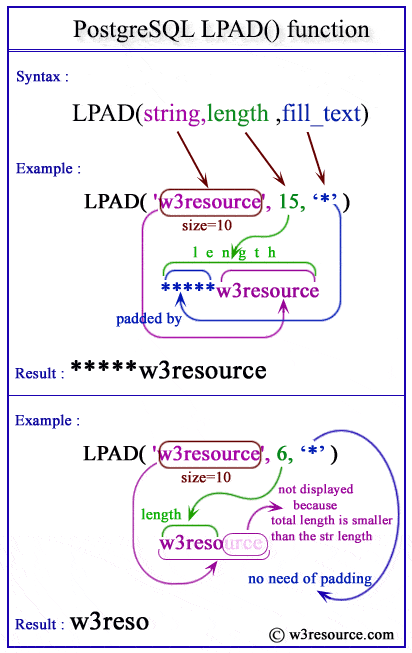The PostgreSQL lpad() function is used to fill up a string of specific length by a substring. If the length of the substring is equal to the remaining length of main string it will fill up properly, if less than the remaining length, the substring will repeat until it is not filling up, if longer than the remaining length or specified length it will be truncated on the right.

Syntax:

`lpad(string,length[,fill_text])`

Parameters:

Name Description Return Type
string A string, which will be filled up by another string. text
length The length of the string, which will be after filled up by substring.. integer
fill_text The substring which will be fill up the sting to length. text

PostgreSQL Version: 9.3

Pictorial Presentation of PostgreSQL LPAD() functionIn the example below, the main string is 'esource' and its length is 7, the substring is 'w3r' of length 3 and the string have to be a length of 10. So, remaining length is 3, and the substring 'w3r' will fill up properly and the result is 'w3resource'.

Code:

``````
```
```

Sample Output:

```    lpad
------------
w3resource
(1 row)
```

Example 2:

In the example below, the main string is 'esource' and its length is 7, the substring is 'w3r' of length 3 and the string have to be a length of 13. So, remaining length is 6, and the substring 'w3r' will repeat two times to fill it up, thus the result is 'w3rw3resource'.

Code:

``````SELECT lpad('esource', 13, 'w3r');
```
```

Sample Output:

```    lpad
---------------
w3rw3resource
(1 row)
```

Example 3:

In the example below, the main string is 'w3resource' and its length is 10, the substring is 'lpad' of length 4 and the string have to be a length of 8. Here, the specified length is smaller than the string, so, instead of lpadding the string will be truncated by two characters from the right side of the string, thus the result is 'w3resour'.

Code:

``````SELECT lpad('w3resource', 8, 'lpad');
```
```

Sample Output:

```  lpad
----------
w3resour
(1 row)
```

Previous: LENGTH function
Next: LTRIM function

﻿